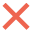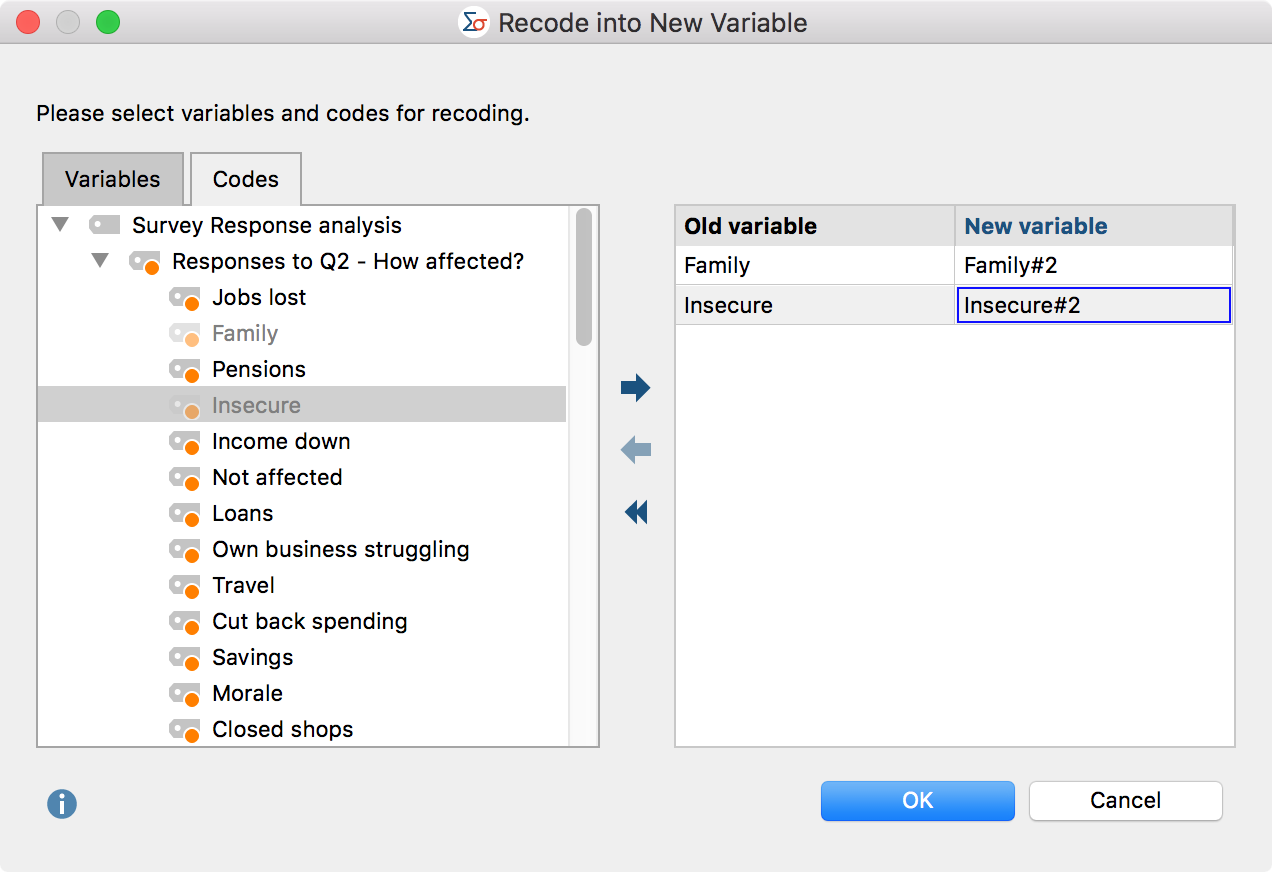# Transform Data – Compute Variables

With MAXQDA Stats you can perform calculations on a selected variable and store the result in a new variable. To begin the calculation, select the function Transform > Compute variable from the Stats main menu. The following window will appear:When calculating variables, proceed as follows:

1. In the upper pane of the window, assign a new variable name and a label if required. If the variable name already exists, MAXQDA will indicate it in red. The newly created variable will automatically be assigned the type “Floating”.
2. In the lower pane “Numerical expression”, assign an evaluation instruction. If the input cannot be evaluated, for example because a bracket or a summand is missing, MAXQDA will indicate so in blue as displayed in the screenshot.

In the “Variable” section, all “Integer” and “Floating point” type variables are listed. All variables are numbered consecutively from “V1” to “Vn”, with the variable name (or the label if present) enclosed in square brackets. When proceeding with the calculation, the identifier must be inputted as displayed in the screenshot above.

Tip: Double-clicking a variable will insert it into the current cursor position, so it does not need to be entered manually.

In the right hand pane, “Functions”, frequently used functions including the applied syntax are listed. Click the function buttons to insert the function in the calculation pane.

 Function Meaning +  –  *  / Add, subtract, multiply, divide ^ Exponent, for example “V1^2” is the variable “V1” squared avg(a, b, …) Arithmetic mean of all variables or arithmetic expressions enclosed in the parentheses (separated by commas). Any number of variables or arithmetic expressions can be enclosed in the parentheses. sum(a, b, …) Sum of all variables or arithmetic expressions enclosed in the parentheses (separated by commas). Any number of variables or arithmetic expressions can be enclosed in the parentheses. sqrt(a) Root of the expression in parentheses round(a) The arithmetic expression in parentheses will be rounded to the next whole number roundn(a, decimals) The arithmetic expression in parentheses will be rounded to the specified number of decimal places

When you click OK, the new variable will be calculated and displayed in the Data Editor.

Hint: If one of the variable values in the calculation instruction is empty or defined as missing, a system-defined missing value will be entered for the result, and the cell will remain empty.

You can also include if-then conditions in the calculation. The following examples illustrate the syntax to be used:

if (V1 > V2) {

V1

}

else {

V2

}

Alternative Syntax:

if (V1 > V2)

V1;

else

V2

## Recode variables

Often when working with datasets there is the desire to recode variables; for example, to reverse the polarity of an item or group multiple variables as a single variable, summarized as, for example, differentiated collected information on schooling for three levels of education. MAXQDA Stats allows recoding of multiple variables or variable values at a time.

### Recode into new variable

Normally variable values are recoded as new variables so that the original value will not be affected. Proceed as follows:

1. Begin the recoding process from the menu function Transform > Recode into new variable.
2. The following window will appear:

3. In the left hand pane, select the variables that you wish to recode. Double-click the desired variable to transfer it to the right-hand pane. Multiple variables can be transferred at a time by holding the Ctrl/cmd key as the variables are selected, then moving them to the right pane with the mouse or by using the blue arrow in the center of the window.

4. For the new variable name, MAXQDA Stats automatically suggests the original name supplemented with a number after a hashtag #. You can modify the name by editing it in the cell.
5. When you click OK, a dialog box will appear for the input of the modified values:

In the “Old value” column, enter the original value and in the “New value” column, enter the target value to be recoded. The image above shows a classic example: the value of the items is reversed, so that the lowest value of 1 becomes the highest value of 4, and vice versa.

Using the selection list in the first column, you can specify a range of values to which a single new value will be assigned. In the selection list, the operators greater than (>); less than (<); greater than or equal to (); or lesser than or equal to () are available. Using the following rows, a variable with a value of 3 can for example be dichotomized, so that in the new variable 0 is displayed for values under 3, and 1 is displayed for values over 3:The button Insert all existing values lists the occurring values for all selected variables, which is especially practical when all values of a variable will be recoded and you wish to immediately begin entering the new values.

Theandsymbols allow you to delete individual or all rows.

6. When you click OK the new variable will be inserted and displayed in the Data Editor. The variable label will appear as the original supplemented with the suffix “recoded” so the recoded variable can be easily recognized. If no label is present, the variable name is used as a label to which “recoded” is added.

MAXQDA Stats proceeds specifically as follows when recoding:

• In case of conflict of the attribution, the lower attribution will be applied. For example, if the first row states “1 to 4” and the second row states “>0 to 3”, 1 will be recoded as 3 and not 4.
• If no new value is specified for an existing value, the old value will be retained.
• Value labels will not be transferred to the new variable, but the definitions for missing values.

### Recode code frequencies per document as new variables

With MAXQDA Stats, you can not only recode variables, but also the code frequencies that were transferred from a MAXQDA project to MAXQDA Stats. If you launched MAXQDA Stats with the data from a MAXQDA project, click the “Code” tab in the dialog box to select individual codes from the familiar code tree.

To recode a code as a new variable, double-click the code or drag it into the right pane of the window using the mouse. You can also use the blue arrow to transfer codes to the right pane.Assuming that you are only interested in whether or not a code has been assigned in a document, the exact number of code frequencies is not relevant, but only whether 0 or a higher number is displayed. In this case the code can be recoded in a single row and stored as a new variable:### Recoding into the same variable

Recoding into the same variable is performed in the same way as recoding into a new variable, from the menu Transform > Recode into same variable. However, for this procedure only variables, not codes, can be selected, and naturally the definition of a new variable name is omitted.